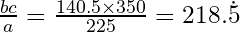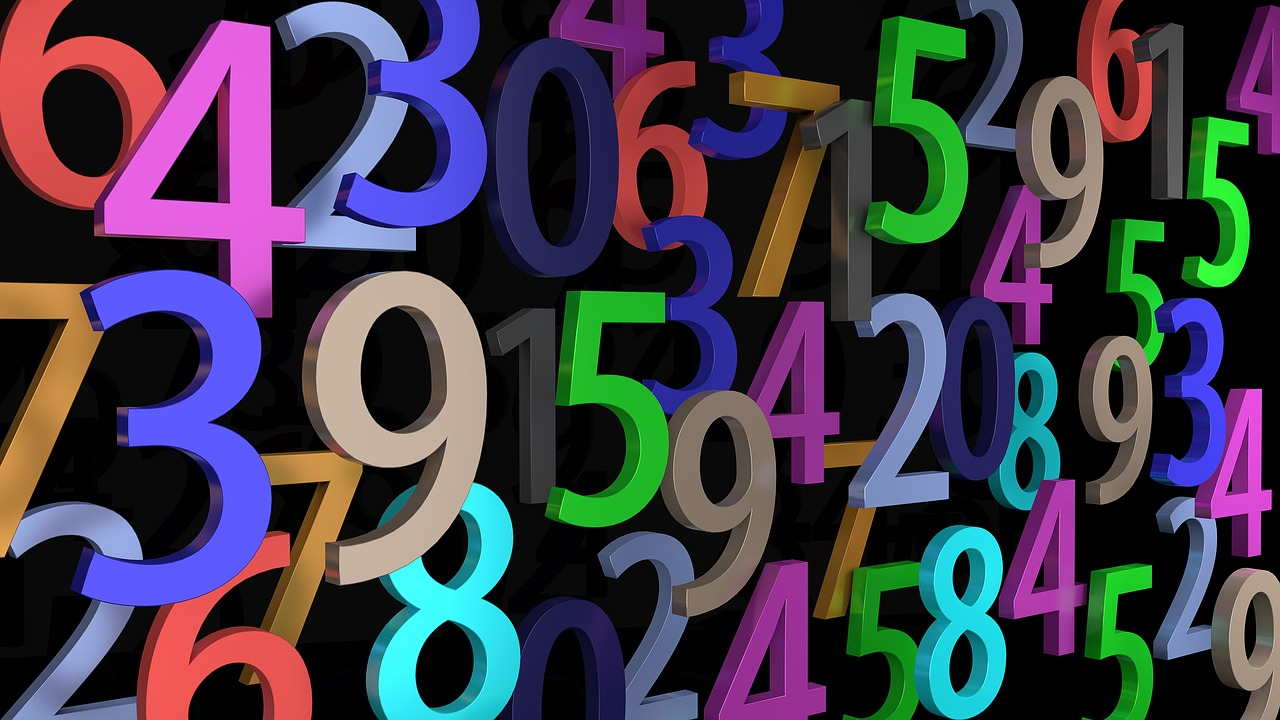# How to work with upper and lower bounds

Once you’ve got to grips with the basic techniques involved in rounding numbers, as covered in my recent blog post, the next step is to deal with upper and lower bounds.

The section down to the embedded video is required for both Foundation and Higher GCSE, but the lower section is mainly just for Higher.

## Identifying upper and lower bounds

A number line can come in handy here. The key is to work out (a) what’s the smallest number that would be rounded up to the number we’re looking at, and (b) when you’d start to round up to the next mark on the scale. For the upper bound, you also need to consider whether the quantity you’re dealing with is discrete or continuous.

### Discrete quantities

If there are 15000 people in a stadium, rounded to the nearest thousand, then you should picture, or sketch, a number line with divisions at intervals of 1000:

The cut-off at which we start to round up to 15000, rather than down to 14000, is half way in between the two values, at 14500, so 14500 is the lower bound.

Similarly, at 15500, we stop rounding down to 15000 and start rounding up to 16000.

Now, since you can only have a whole number of people – it’s a discrete quantity – the maximum number of people that will round down to 15000 is one less than 15500, i.e. 15499.

Therefore the upper bound is 15499.

So 15000 to the nearest thousand could be any integer from 14500 up to and including 15499.

What if the number were 15000 to the nearest hundred?

This time the divisions on the scale will only be at intervals of 100, so the next one down will be 14900 and the next one up will be 15100. What are the cutoffs this time? So what will the upper and lower bounds be?

The lower bound will be 14950 and the upper bound will be one less than 15050, i.e. 15049.

So 15000 to the nearest hundred could be any integer from 14950 up to and including 15049.

If you had a collection of 350 china elephants, rounded to the nearest 50, what would be the upper and lower bounds? Answers at the bottom of the page.

### Continuous quantities

If the 15000 were a continuous quantity, for example a car had covered 15000 miles to the nearest thousand miles, then the lower bound would still be the same, but you’d round 15499.99… miles down to 15000.

Since there is no gap between the largest number you’d round down to 15000 and the smallest number you’d round up to the next thousand, the upper bound for 15000 is the same as the lower bound for 16000, i.e. 15500.

So 15000 to the nearest thousand could be any value from 14500 up to but not including 15500.

Similarly, if you were rounding to the nearest hundred miles then the lower and upper bounds would be 14950 and 15050 respectively.

So 15000 to the nearest hundred could be any value from 14950 up to but not including 15050.

Questions for you to try: (Answers at the bottom of the page)

1. If a footpath was 260 m long, measured to the nearest 20 m, what would be the upper and lower bounds for its length?
2. If a vehicle weighs 1200 kg to 2 significant figures, what are the upper and lower bounds for its mass?

## Error intervals

An error interval is simply the lower and upper bounds expressed as a double-ended inequality.

For the examples in the last section, they look like this:

### Discrete quantities

Remember, with discrete data you include the upper bound; if n = 15000 to the nearest thousand then n could be any integer from 14500 up to and including 15499, or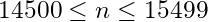If n = 15000 to the nearest hundred then n could be any integer from 14950 up to and including 15049, orWhat would the error interval look like for 350 china elephants rounded to the nearest 50? Answer at the bottom of the page.

### Continuous quantities

If n = 15000 to the nearest thousand then n could be any value from 14500 up to but not including 15500, or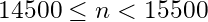If n = 15000 to the nearest hundred then n could be any value from 14950 up to but not including 15050, or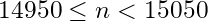What would the error interval look like for a footpath of length 260 m rounded to the nearest 20 m? Answer at the bottom of the page.

## Truncation

Truncation is a kind of “lazy rounding”: you don’t bother to decide whether to round up or down, you just chop the number off at the specified place value (and pad out with zeroes if necessary).

So 15000 truncated at the thousands column could be any number starting with 15 thousand…

… so the lower bound is 15000 and the upper bound is 16000 for continuous data, or 15999 for discrete (if counted in ones).

What would the error interval look like for a mass of 7 kg truncated to the nearest kg? Answer at the bottom of the page.

## Video lesson

Here’s a video lesson from the Grade 4 Essentials for GCSE Maths course, covering the same material as the Foundation content of this article. Take a look at the course if you feel you’d benefit from a bit of extra support to hit that magic Grade 4.

## Using bounds in calculations

When you use bounds in calculations, it can take some thought to decide which bound is going to give the result you want.

### Example 1

A rectangle has a length of 16 cm, measured to the nearest cm, and a width of 7.5 cm, measured to the nearest 0.5 cm. What are the upper and lower bounds for the area and perimeter of the shape?

For 16 cm, LB = 15.5 cm and UB = 16.5 cm
For 7.5 cm, LB = 7.25 cm and UB = 7.75 cm

To find the area we multiply. To get the biggest possible answer we need to multiply both upper bounds, and for the smallest possible answer we need to multiply both lower bounds.
So max area = 16.5 x 7.75 = 127.875 cm²
and min area = 15.5 x 7.25 = 112.375 cm²

What if you were asked to give the area of the rectangle “to an appropriate degree of accuracy”? (With a question like this you probably wouldn’t be explicitly asked to find the upper and lower bounds of the area, but it would be expected.)

The initial measurements are only given to 2 significant figures so your final answer can’t possibly be more accurate than that. But if you rounded 127.875 to 2 s.f. then you’d get 130, and 112.375 rounded to 2 s.f. would be 110. You need a single value that covers the full range of possible areas, so you’d have to reduce the number of significant figures to one. Therefore the answer would have to be just 100 cm²… which of course wouldn’t be very helpful, as it would imply that the area could be anything between 50 and 150 cm²!

In fact that’s why, when working with acceleration due to gravity, mathematicians normally use 9.8 m/s² while physicists favour 9.81. The actual value can range from 9.780 at the equator to 9.832 at the poles (so you’re heavier at the North Pole that you are at the equator). Both of these extremes round to 9.8 so that’s the value favoured by mathematicians: it’s always correct, but it may not be precise enough to be very useful in practical terms!

Physicists, on the other hand, are more pragmatic and prefer to take the mean of the extremes, which is 9.81 to 3 s.f. and gives a good approximation for most places on Earth. If they want a result that’s specific to a particular location then they can always re-do the calculation with an appropriate value for that location.

Now your turn: Work out the upper and lower bounds for the perimeter. Answers at the bottom of the page.

### Example 2

Given that
a = 230 to 2 s.f.
b = 140 to 3 s.f.
c = 300 to 1 s.f.
find the maximum possible value of

(a)(b)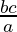These are a bit trickier. When you’re subtracting, you’ll get a bigger difference if you subtract a small number from a big one than vice versa. So to get the biggest possible difference, we need to start with the upper bound of one value and subtract the lower bound of the other value.

Similarly, when you’re dividing, if you divide by a bigger number then you’re going to get a smaller answer. In this case we’re looking for a big answer, so we want the biggest possible number on the top of the formula and the smallest possible number on the bottom.

What are the upper and lower bounds for each of a, b and c?
a: LB = 225; UB = 235
b: LB = 139.5; UB = 140.5
c: LB = 250; UB = 350

So for (a) the answer will be 235 x 140.5 – 250 = 132.07.

What will the answer be for (b)? Scroll down to the bottom to check.

So that covers the topic on upper and lower bounds. If you’ve found this post helpful then please share it, and if you’d like to receive my monthly email updates then please subscribe to my mailing list using the link in the footer of this page.

China elephants: LB = 325; UB = 374

Footpath length: LB = 250 m; UB = 270 m
Vehicle mass: the 2nd s.f. is the hundreds column so it’s rounded to the nearest 100.
Therefore LB = 1150 kg; UB = 1250 kg

Error intervals: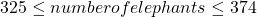;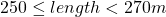Truncation: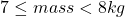Rectangle perimeter: LB = 45.5 cm; UB = 48.5 cm

Calculation: